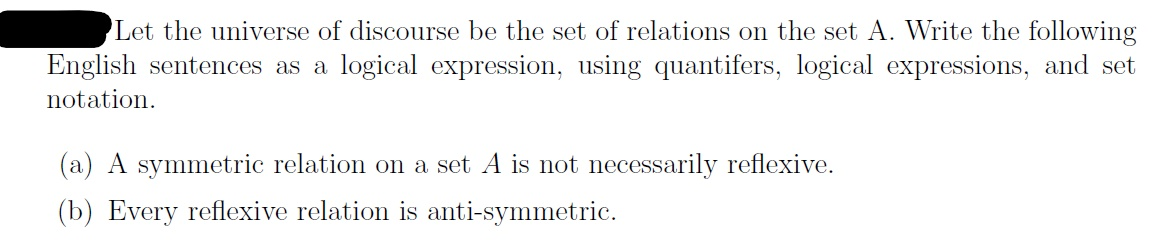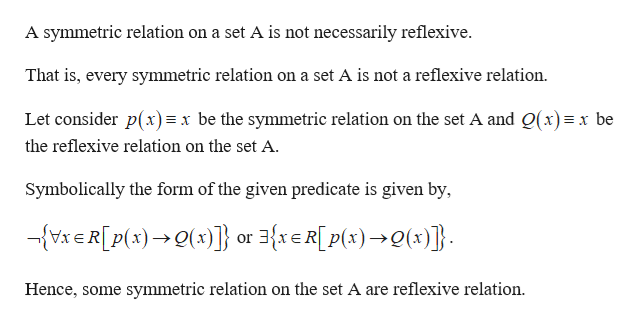# Let the universe of discourse be the set of relations on the set A. Write the followingEnglish sentences as a logical expression, using quantifers, logical expressions, and setnotation(a) A symmetric relation on a set A is not necessarily reflexive(b) Every reflexive relation is anti-symmetric

Question
68 views

thank youhelp_outlineImage TranscriptioncloseLet the universe of discourse be the set of relations on the set A. Write the following English sentences as a logical expression, using quantifers, logical expressions, and set notation (a) A symmetric relation on a set A is not necessarily reflexive (b) Every reflexive relation is anti-symmetric fullscreen
check_circle

star
star
star
star
star
1 Rating
Step 1

Given the universe of discourse be the set of relation on the set A.

Let the universe o...help_outlineImage TranscriptioncloseA symmetric relation on a set A is not necessarily reflexive That is, every symmetric relation on a set A is not a reflexive relation Let consider p(x)= x be the symmetric relation on the set A and Q(x)x be the reflexive relation on the set A Symbolically the form of the given predicate is given by, - ) or xeR[p()l)}. Hence, some symmetric relation on the set A are reflexive relation fullscreen

### Want to see the full answer?

See Solution

#### Want to see this answer and more?

Solutions are written by subject experts who are available 24/7. Questions are typically answered within 1 hour.*

See Solution
*Response times may vary by subject and question.
Tagged in

### Math